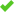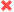# 使您的网站多语言## 多语言网站变得容易

GTranslate可以翻译任何HTML网站，并使其成为多语言。 它将帮助您增加国际流量，吸引全球观众，开拓新市场。

### 尝试使用免费的15日试验GTranslate

• 0+ 十亿 翻译页面服务
• 0+ 百万 免费插件下载
• 0+ 兆字节 翻译过去一个月
• 0 活跃客户### 搜索引擎索引### 搜索引擎友好### 机器翻译### 翻译编辑### 使用统计### 语言托管### 网址翻译## 15日无风险试用

### Free

\$ 0• 语言选择器小部件
•所有语言
•机器翻译
•无限的单词和综合浏览量
•搜索引擎索引
•编辑翻译
•网址翻译
•语言托管

### Custom

\$ 5
.99 /月### Startup

\$ 14
.99 /月• 翻译交付网络
•所有语言
•神经翻译
•无限的单词和综合浏览量
•搜索引擎索引
•编辑翻译
•网址翻译
•语言托管

### Enterprise

\$ 34
.99 /月• 翻译交付网络
•所有语言
•神经翻译
•无限的单词和综合浏览量
•搜索引擎索引
•编辑翻译
•网址翻译
•语言托管• 南非荷兰语 <-> 英语
• 阿尔巴尼亚人 <-> 英语
• 阿姆哈拉语 <-> 英语
• 阿拉伯语 <-> 英语
• 亚美尼亚 <-> 英语
• Azeerbaijani <-> 英语
• 巴斯克 <-> 英语
• 孟加拉 <-> 英语
• 波斯尼亚 <-> 英语
• 保加利亚语 <-> 英语
• 加泰罗尼亚 <-> 英语
• 宿务 <-> 英语
• 简体中文 <-> 英语
• 中国（繁体） <-> 英语
• 科西嘉 <-> 英语
• 克罗地亚 <-> 英语
• 捷克 <-> 英语
• 丹麦语 <-> 英语
• 荷兰人 <-> 英语
• 爱沙尼亚语 <-> 英语
• 芬兰 <-> 英语
• 法文 <-> 英语
• 加利亚西语 <-> 英语
• 格鲁吉亚语 <-> 英语
• 德语 <-> 英语
• 希腊语 <-> 英语
• 古吉拉特语 <-> 英语
• 希伯来语 <-> 英语
• 印地语 <-> 英语
• 匈牙利 <-> 英语
• 北日耳曼语 <-> 英语
• 印度尼西亚人 <-> 英语
• 意大利语 <-> 英语
• 日语 <-> 英语
• 爪哇 <-> 英语
• 卡纳达语 <-> 英语
• 哈萨克人 <-> 英语
• 高棉 <-> 英语
• 韩语 <-> 英语
• 库尔德人 <-> 英语
• 拉脱维亚 <-> 英语
• 立陶宛 <-> 英语
• 马其顿 <-> 英语
• 马来语 <-> 英语
• 马拉雅拉姆语 <-> 英语
• 马拉 <-> 英语
• 蒙古人 <-> 英语
• 挪威语 <-> 英语
• Nyanja（奇切瓦） <-> 英语
• 普什图语 <-> 英语
• 波斯语 <-> 英语
• 波兰语 <-> 英语
• 葡萄牙语 <-> 英语
• 旁遮普 <-> 英语
• 罗马尼亚 <-> 英语
• 俄语 <-> 英语
• 塞尔维亚 <-> 英语
• 塞索托语 <-> 英语
• 绍纳语 <-> 英语
• 信德 <-> 英语
• 僧伽罗人 <-> 英语
• 斯洛伐克 <-> 英语
• 斯洛文尼亚 <-> 英语
• 西班牙语 <-> 英语
• 斯瓦希里 <-> 英语
• 瑞典语 <-> 英语
• 菲律宾语（菲律宾语） <-> 英语
• 泰米尔人 <-> 英语
• 泰卢固语 <-> 英语
• 泰国人 <-> 英语
• 土耳其 <-> 英语
• 乌克兰 <-> 英语
• 乌尔都语 <-> 英语
• 乌兹别克 <-> 英语
• 越南人 <-> 英语
• 科萨 <-> 英语
• 祖鲁 <-> 英语• Custom
• Enterprise
• 清除地理位置定位
• 最适合搜索引擎优化
• 容易分离的场地
• 没有服务器要求
• 昂贵
• 严格的ccTLD要求（有时）

• Custom
• Startup
• Enterprise
• 更好的地理位置定位
• 对SEO很好
• 容易分离的场地
• 没有服务器要求
• 用户可能无法从URL中识别地理位置定位

• Custom
• Startup
• Enterprise
• 良好的地理位置定位
• 对SEO很好
• 用户可能无法从URL中识别地理位置定位
• 没有分离的网站
• 您的服务器需要PHP
URL参数 example.com?lang=de 不可用
• 不建议
• 基于URL的分段困难
• 用户可能无法从URL中识别地理位置定位
• 快速设置
• 没有服务器要求
• 没有在搜索引擎索引
• 没有SEO优势GTranslate TDN技术是一种基于云的网站翻译方法。 无需更改您的环境并安装软件来本地化您的网站。 您的多语言网站可以在5分钟内运行。15日免费试用如何工作？